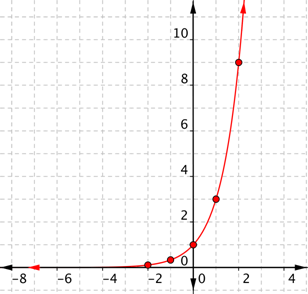# Elementary Functions / Non Elementary Functions

## What are Elementary Functions?An exponential function is one example of an elementary function.An elementary function is a real function built from basic building blocks: constants, sums, differences, roots, quotients, powers, exponential functions, logarithmic functions, polynomial functions, trigonometric functions and inverse trigonometric functions.

Elementary functions are those familiar functions commonly used throughout algebra and calculus, and in most scientific and economic applications. Whether or not a function is “elementary” is usually arrived at by consensus, so now and then you may come across a function that is described as “elementary” by an author, but it might not be universally agreed upon.

## A More Mathematical Definition

The term “elementary” is often used loosely, applying to commonly used functions, functions of one variable, or those made up from a list of common functions. In his 2016 paper titled Definition and Some Properties of Elementary Functions, Angel S. Muleshkov argues for a more mathematical definition, defining the following eight functions as the “fundamental elementary functions of a real variable”:

1. f1 (x) = c, c ∈ ℝ, with domain D ⊆ ℝ
2. f2 (x) = x, with domain D ⊆ ℝ
3. f3 (x) = 1/x, with domain D ⊆ ℝ\{0}
4. f4 (x) = n√ x, n ∈ ℕ, if n/2, ∈ ℕ then domain D ⊆ [0, +∞), and if (n + 1)/
2 ∈ ℕ then domain D ⊆ ℝ
5. f5 (x) = sin x, with domain D ⊆ ℝ
6. f6 (x) = ex, with domain D ⊆ ℝ
7. f7 (x) = ln x, with domain D ⊆ (0, +∞)
8. f8 (x) = arccos x, with domain D ⊆ [−1, 1]

Notation:

## Derivative and Integral of Elementary Functions

The derivative of an elementary function is also an elementary function. However, the integral of an elementary function may, or may not be, elementary.

## Elementary Function of Class n

The term “elementary functions of class n” relate to those functions containing complex variables. For example, an elementary function of class 0 is an algebraic function made up of a finite number of complex variables, and ez and log(z) are elementary functions of class 1.

## What is a Non Elementary Function?

Any function that isn’t elementary is non elementary. A precise mathematical definition doesn’t exist; The definition is one of exclusion (i.e. anything that isn’t elementary is non-elementary).

Important non elementary functions include the gamma function, Lambert W-function, and many others.

## References

Clark, D. (1999). Dictionary of Analysis, Calculus, and Differential Equations. CRC Press.
Keisler, H. (2013). Elementary Calculus: An Infinitesimal Approach, Courier Corporation.
Muleshkov, A. (2016). Definition and Some Properties of Elementary Functions. Retrieved December 1, 2019 from: http://muleshko.faculty.unlv.edu//handouts/Elementary%20Functions%20(1).pdf
Ritger, P. & Rose, N. (2000). Differential Equations with Applications. Courier Corporation.

CITE THIS AS:
Stephanie Glen. "Elementary Functions / Non Elementary Functions" From StatisticsHowTo.com: Elementary Statistics for the rest of us! https://www.statisticshowto.com/elementary-functions/